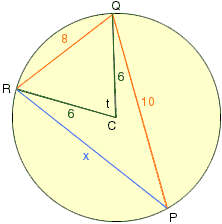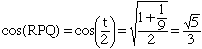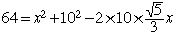Name: Marko Who is asking: Student Level: Secondary Question: In a circle of radius 6, a triangle PQR is drawn having QR = 8 and PQ = 10. Determine the length of PR Hi Marko, In the diagram below, t is the angle measurement of angle RCQ where C is the center of the circle. Use the Law of Cosines with triangle CQR to find cos(t) = 72-64/72 = 1/9The angle RPQ has angle measurement  t/2 and henceLet x be the length of the side RP then, using the Law of Cosines with triangle RPQ,The general quadratic can now be used to find x. Cheers, Chris Go to Math Central